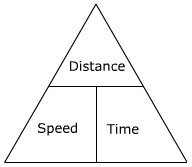QuestionAnswers

# A car travels the distance from Mumbai to Goa in 3 hours at a speed of 60 km/h and returns from Goa to Mumbai speed of 40 km/h. How long did it take to reach Mumbai?Verified
128.7k+ views
Hint:Distance Speed Time Formula
Speed is a measure of how quickly an object moves from one place to another. It is equal to the distance travelled divided by the time. It is possible to find any of these three values using the other two. This picture is helpful:The positions of the words in the triangle show where they need to go in the equations. To find the speed, distance is over time in the triangle, so speed is distance divided by time. To find distance, speed is beside time, so distance is speed multiplied by time.
$Speed = \dfrac{{dis\tan ce}}{{time}} \\ time = \dfrac{{dis\tan ce}}{{Speed}} \\ dis\tan ce = speed{\rm X}time \\$

Firstly we will find the distance of the journey between Mumbai to Goa he has travelled.
Speed = 60 Km/h
Time = 3 hours
Distance = Speed X Time
$Distance = 60{\rm X}3 = 180Km$
Secondly the distance of the journey between Mumbai to Goa and Journey between Goa to Mumbai he has travelled are the same.
So, Distance remains same = 180 Km
Speed = 40 Km/h
So, Time = $\dfrac{{distance}}{{time}} = \dfrac{{180}}{{40}} = 4.5hours$

Time required to reach back to Mumbai is 4.5 hours.

Note:Speed, distance, and time problems ask to solve for one of the three variables given certain information. In these problems, objects are moving at either constant speeds or average speeds.
Most problems will give values for two variables and ask for the third.